UPSC  >  Important Formulas: Number System

# Important Formulas: Number System - CSAT Preparation - UPSC

 Table of contentsImportant Formulas in AlgebraVisual Representation of Some Formulas in AlgebraImportant Formulas in Number SystemMethod Related to Example 7 to 10DivisibilityHCF and LCMNumber System is an all-time favourite with the competitive exam setters as well as the students. The topic is known for intriguing conceptual problems that test the best brains.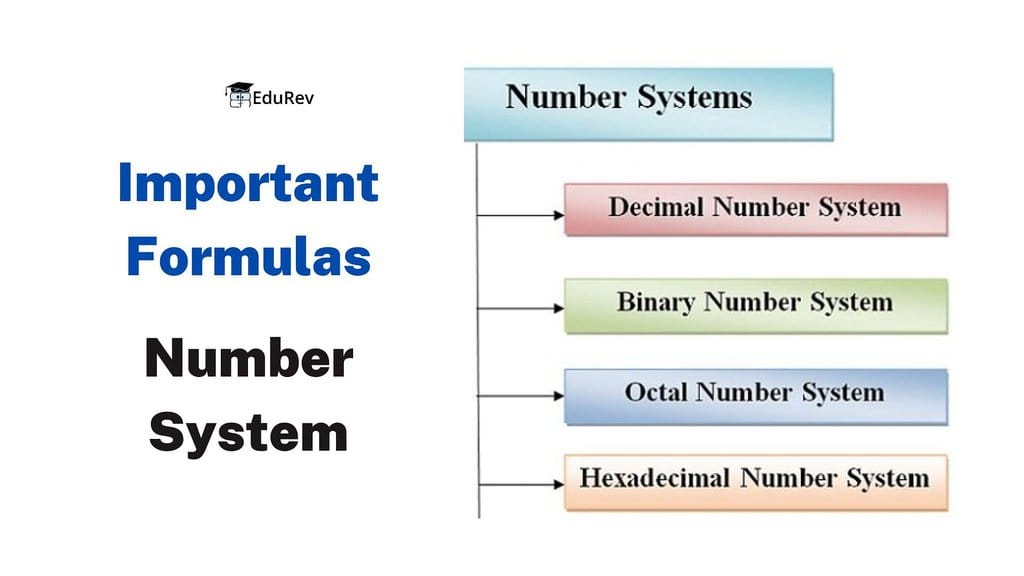• It becomes important that candidates are well versed with the basic formulas required for solving questions with higher accuracy and in the least time possible.
• Here is a list of Important Formulae that candidates need to master to crack the exam successfully.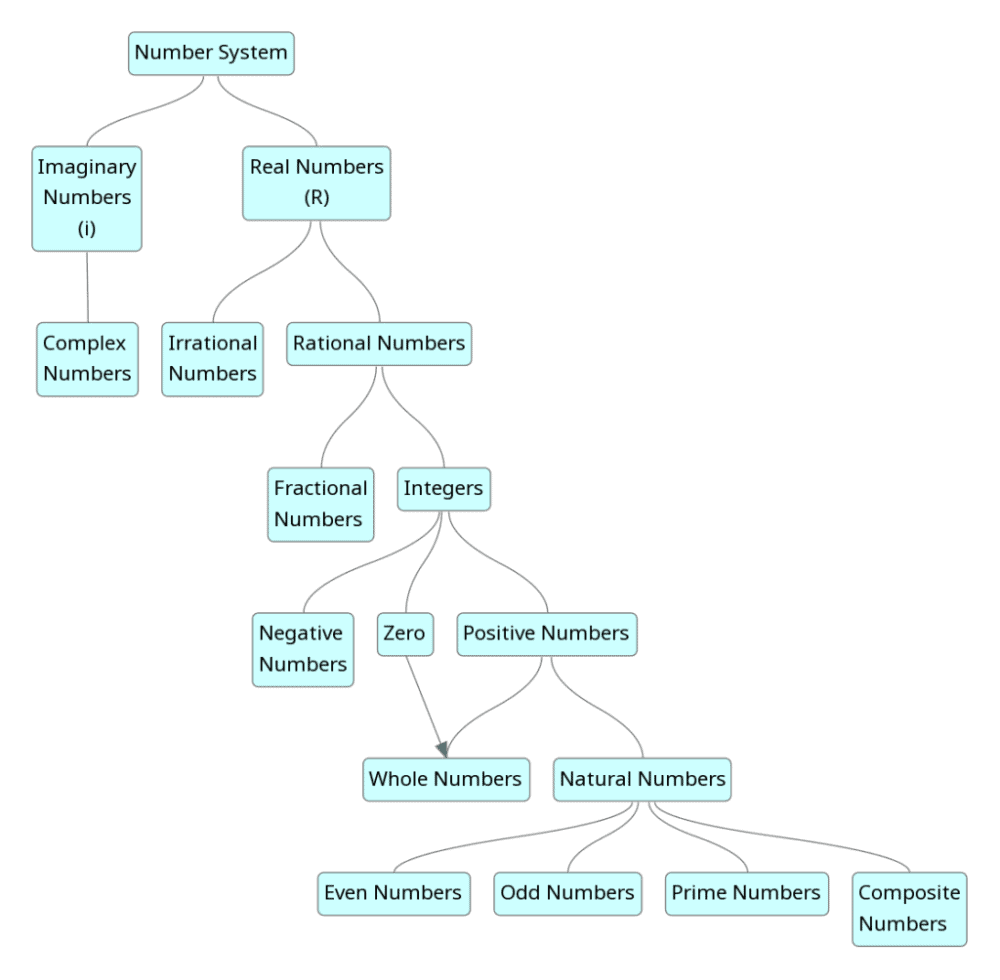## Important Formulas in Algebra

Algebra is a branch of mathematics that substitutes letters for numbers. An algebraic equation depicts a scale, what is done on one side of the scale with a number is also done to either side of the scale.

• (a + b)(a - b) = (a2 - b2)
• (a + b)2 = (a2 + b2 + 2ab)
• (a - b)2 = (a2 + b2 - 2ab)
• (a + b + c)2 = a2 + b2 + c2 + 2(ab + bc + ca)
• (a3 + b3) = (a + b)(a2 - ab + b2)
• (a3 - b3) = (a - b)(a2 + ab + b2)
• (a3 + b3 + c3 - 3abc) = (a + b + c)(a2 + b2 + c2 - ab - bc - ac)
⇒ When a + b + c = 0, then a3 + b3 + c3 = 3abc
• (a + b)= an + (nC1)an-1b + (nC2)an-2b² + … + (nCn-1)abn-1 + bn

Question for Important Formulas: Number System
Try yourself:What is the square of 109?

## Visual Representation of Some Formulas in Algebra

• (a + b)2 = a2 + b2 + 2ab
The (a + b)formula is the algebraic identity used to find the square of the sum of two numbers. To find the formula of the binomial in the form (a + b)2, we will just multiply (a + b) (a + b).
(a + b)2 = (a + b)(a + b)
= a+ ab + ba + b2
= a2 + 2ab + b2
Therefore, (a + b)2 formula is: (a + b)2 = a2 + 2ab + b2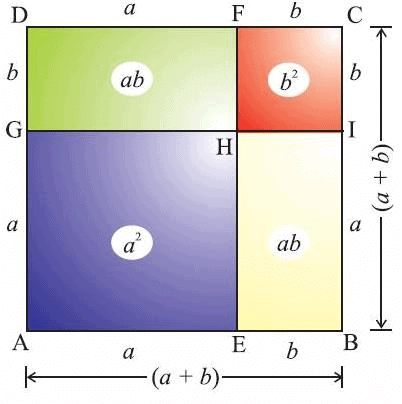• (a + b + c)2 = a2 + b2 + c2 + 2ab + 2bc + 2ca
The (a + b + c)2 formula is used to find the sum of squares of three numbers without actually calculating the squares. (a + b + c)2 formula is one of the major algebraic identities. To derive the expansion of (a + b + c)2 formula we just multiply (a + b + c) by itself to get (a + b + c)2.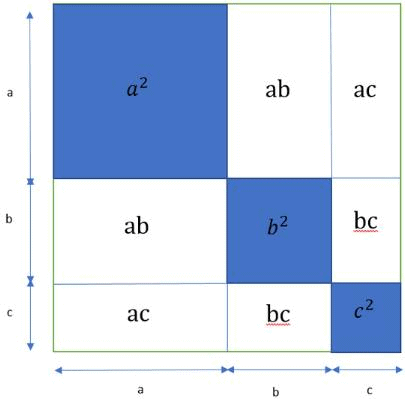## Important Formulas in Number System

• 1 + 2 + 3 + 4 + 5 + … + n = n(n + 1)/2
• (1² + 2² + 3² + ….. + n²) = n ( n + 1 ) (2n + 1) / 6
• (1³ + 2³ + 3³ + ….. + n³) = (n(n + 1)/ 2)²
• Sum of first n odd numbers = n²
• Sum of first n even numbers = n (n + 1)
• Highest power of n in m! is [m/n] + [m/n2] + [m/n3] +….. where, [x] is the greatest integer less than or equal to x.

Let's have a look at some solved examples:

Example 1: Find the highest power of 7 in 100!.

Highest power of 7 in 100! = [100/7] + [100/49] = 16

• To find the number of zeroes in n! find the highest power of 5 in n!

Example 2: What is the number of trailing zeroes in 23!?

[23/5] = 4. It is less than 5, so we stop here. The answer is 4.

•  If all possible permutations of n distinct digits are added together with the sum
= (n-1)! * (sum of n digits) * (11111… n times)

Example 3: What would be the sum of all the numbers which can be formed by using the digits 1, 3, 5, 7 all at a time and which have no digits repeated?

The sum of the numbers formed by taking all the given n digits is (sum of all the n digits) * (n - 1)! * (111…..n times).
Here n = 4, and Sum of 4 digits = 16
The sum of all the numbers which can be formed by using the digits 1, 3, 5, 7= (16) * (4 – 1)! * ( 1111) = 16 * 3! * 1111

• If the number can be represented as N = ap∗ b∗ cr. Then, the number of factors of N is (p+1) * (q+1) * (r+1)
• Sum of all factors: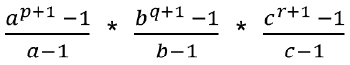Question for Important Formulas: Number System
Try yourself:How many factors does 60 has?

Example 4: Find the number of factors of 98 and also find the sum and product of all factors.

First, write the number 98 into prime factorization.
i.e. 98 = 2 × 49 = 2 × 7 × 7 = 21 x 72
Here  A = 2, B = 7, p = 1, q = 2
Number of factors for 98 = (p + 1)(q +1) = 2 × 3 = 6
Sum of all factors of 98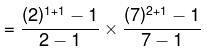= 3 × 57 = 171
Product of all factors of number 98 = (98)6/2 = 941192

Example 5: Find the number of odd, even, perfect square, perfect cube factors of 4500.

4500 = 45 × 100 = 9 × 5 × 10 × 10 = 3 × 3 × 5 × 5 × 2 × 5 × 2 = 22 × 32 × 53
Here, consider A = 2 , B = 3 , C = 5 , p= 2 , q = 2 and r = 3
Here identifying that odd number are 3 and 5.
Numbers of odd factors of number 4500 = (q + 1) (r + 1) = 3 × 4 = 12
∴ Total number of factors = (p + 1)(q +1)(r +1) = 3 × 3 × 4 =36
Numbers of even factors of number = (Total number of factors – Numbers of  odd factors) = 36 – 12 = 24
Number of perfect square factors of number 4500 = 2 x 2 x 2 = 8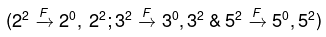Number of perfect cube factors of number 4500 = 1 x 1 x 2 = 2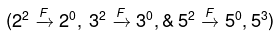• If the number of factors is odd, then N is a perfect square.
• If there are n factors, then the number of pairs of factors would be n/2. If N is a perfect square, then the number of pairs (including the square root) is (n+1)/2.

Example 6: In how many ways you can express 36 as the product of two of its factors?

Prime factorization of 36 i.e. we write 36 = 22 × 32
Number of factors of 36 will be (2+1)(2+1) = 9
(i.e. factors are 1, 2,3, 4, 6, 9, 12, 18, 36).
Since we are asked the total number of ways hence we include the square root of 36 i.e. 6 as well.
Thus the number of ways you can express 36 as the product of two of its factors is (9+1)/2 = 5.

• If the number can be expressed as N = 2p ∗ aq ∗ br . . . where the power of 2 is p and a, b are prime numbers then:
(i) The number of even factors of N = p (1 + q) (1 + r) . . .
(ii) The number of odd factors of N = (1 + q) (1 + r)…
• When we are asked to calculate how many positive integral solutions are possible for the equation X2– Y2= N, there can be 4 cases.
• Case 1: N is an odd number and not a perfect square
The total number of positive integral solutions will be = (Total number of factors of N)/2

How many positive integral solutions are possible for the equation X- Y2 = N?
When we are asked to calculate how many positive integral solutions are possible for the equation X2 - Y2 = N, there can be 4 cases.
Case 1: N is an odd number and not a perfect square
Case 2: N is an odd number and a perfect square
Case 3: N is an even number and not a perfect square
Case 4: N is an even number and a perfect square

Example 7: How many positive integral solutions are possible for the equation X–  Y= 135?

The total number of factors of 135 = 1, 3, 5, 9, 15, 27, 45 and 135 is 8.
So, total number of positive integral solutions = 8/2 = 4.

• Case 2: N is an odd number and a perfect square
The total number of positive integral solutions will be = [(Total number of factors of N) – 1]/ 2

Example 8: How many positive integral solutions are possible for the equation X2– Y= 121?

The total number of factors of 121 = 1, 11 and 121 is 3.
So, the total number of positive integral solutions = (3-1)/2 = 1

• Case 3: N is an even number and not a perfect square
The total number of positive integral solutions will be = [Total number of factors of (N/4)] / 2

Example 9: How many positive integral solutions are possible for the equation X2– Y= 160?

Total number of factors of 40 = 1, 2, 4, 5, 8, 10, 20 and 40 is 8 (as N = 160 and N/4 = 40)
So, total number of positive integral solutions = 8/2 = 4

• Case 4: N is an even number and a perfect square
The total number of positive integral solutions will be = {[Total number of factors of (N/4)] – 1 } / 2

Example 10: How many positive integral solutions are possible for the equation X2– Y2= 256?

The total number of factors of 64 = 1, 2, 4, 8, 16, 32 and 64 is 7 (as N = 256 and N/4 = 64)
So, (7-1)/2 = 3 positive integral solutions.

EduRev Tip: A number can be written as the sum of two squares while including prime factors of the form 4k + 3 as long as those prime factors are raised to an even power.

• The number of digits in ab = [b logm(a)] + 1; where m is the base of the number and [.] denotes the greatest integer function.
• Even a number that is not a multiple of 4, can never be expressed as a difference of 2 perfect squares.
• Basic summation properties:
(i) Sum of first n odd numbers is n2
(ii) Sum of first n even numbers is n(n + 1)
(iii) 1 + 2 + 3 + 4 + 5 + … + n = n(n + 1)/2
(iv) (1² + 2² + 3² + ….. + n²) = n ( n + 1 ) (2n + 1) / 6
(v) (1³ + 2³ + 3³ + ….. + n³) = (n(n + 1)/ 2)²
• The product of the factors of N is given by Na/2, where 'a' is the number of factors.
• The last two digits of a2, (50 – a)2, (50 + a)2, (100 – a)2 . . . . . are same. Every number can be written as (50n ± x), where x is a number from 0 to 25. Hereby 50n, it just means any multiple of 50 i.e. 0, 50, 100, 150, ……
0 to 25 = (0 to 25) itself.
25 to 50 = 50 – (25 to 0)
50 to 75 = 50 + (0 to 25)
75 to 100 = 100 – (25 to 0)
100 to 125 = 100 + (0 to 25)
125 to 150 = 150 – (25 to 0) and so on.

But how does this help us in finding the last two digits of any square?
(50n ± x)2 = 2500n2 ± 100nx + x2
The last two digits of each of 2500n2 and 100nx will be 00. Thus the last two digits of the RHS, and hence of the LHS, will be the last two digits of x2.

Example 11: What are the last two digits of 2682?

268 = 50 × 5 + 18.
Thus, the last two digits of 2682 will be same as the last two digits of 182 i.e. 24.

Example 12: What are the last two digits of 2782?

While you can consider 278 = 50 × 5 + 28, it will consider
278 = 50 × 6 – 22, to be x in the range 0 to 25.
Thus, the last two digits of 2782 will be the same as the last two digits of 222 i.e. 84.

•  If the number is written as 210n:
(i) When n is odd, the last 2 digits are 24.
(ii) When n is even, the last 2 digits are 76.

## Divisibility

• Divisibility by 2 → A number that is even or a number whose last digit is an even number i.e. 0, 2, 4, 6, and 8.
• Divisibility by 3 → The sum of all the digits of the number should be divisible by 3.
• Divisibility by 4 → Number formed by the last two digits of the number should be divisible by 4 or should be 00.
• Divisibility by 5 → Numbers having 0 or 5 as their ones place digit.
• Divisibility by 6 → A number that is divisible by both 2 and 3.
• Divisibility by 7 → Subtracting twice the last digit of the number from the remaining digits gives a multiple of 7.
• Divisibility by 8 → Number formed by the last three digits of the number should be divisible by 8 or should be 000.
• Divisibility by 9 → The sum of all the digits of the number should be divisible by 9.
• Divisibility by 10 → Divisibility rule for 10 states that any number whose last digit is 0, is divisible by 10.
• Divisibility by 11 → The difference of the sums of the alternative digits of a number is divisible by 11.
• Divisibility by 12 → A number that is divisible by both 3 and 4.
• Divisibility by 13 → For any given number, to check if it is divisible by 13, we have to add four times of the last digit of the number to the remaining number and repeat the process until you get a two-digit number.  Now check if that two-digit number is divisible by 13 or not. If it is divisible, then the given number is divisible by 13.
• Divisibility by 14→ A number divisible by both 2 and 7.
• Divisibility by 16 → Last four-digit divisible by 16
• Divisibility by 27 → Sum of blocks of 3 (taken a right to left) divisible by 27

### Let's have a look at solved examples of Divisibility

Q.1. Is 7248 is divisible (i) by 4, (ii) by 2, and (iii) by 8?

(i) The number 7248 has 48 on its extreme right side which is exactly divisible by 4. When we divide 48 by 4 we get 12.

Therefore, 7248 is divisible by 4.

(ii) The number 7248 has 8 on its unit place which is an even number so, 7248 is divisible by 2.

(iii) 7248 is divisible by 8 as 7248 has 248 at its hundred place, tens place and unit place which is exactly divisible by 8.

Q.2. A number is divisible by 4 and 12. Is it necessary that it will be divisible by 48? Give another example in support of your answer.

Answer: 48 = 4 × 12 but 4 and 12 are not co-prime.

Therefore, it is not necessary that the number will be divisible by 48.

Let us consider the number 72 for an example

72 ÷ 4 = 18, so 72 is divisible by 4.

72 ÷ 12 = 6, so 72 is divisible by 12.

But 72 is not divisible by 48.

Q.3.  Without actual division, find if 235932 is divisible (i) by 4 and (ii) 8.

(i) The number formed by the last two digits on the extreme right side of 235932 is 32

32 ÷ 4 = 8, i.e. 32 is divisible by 4.

Therefore, 235932 is divisible by 4.

(ii) The number formed by the last three digits on the extreme right side of 235932 is 932

But 932 is not divisible by 8.

Therefore, 235932 is not divisible by 8.

Q.4. Check whether 998 is divisible by 9.

According to the rule, if the sum of the digits in a number is a multiple of 9, then it is divisible by 9.
Sum of the digits in 998: 9 + 9 + 8  =  26
26 is not a multiple of 9
So, 998 is not divisible by 9.

Q.5. Check whether 1782 is divisible by 11.

According to the rule, in a number, if the sum of the digits in odd places and sum of the digits in even places are equal or they differ by a number divisible by 11, then the number is divisible by 11.
In 1782, the sum of the digits in odd places:
1 + 8  =  9
In 1782, the sum of the digits in even places:
7 + 2  =  9
In 1782, the sum of the digits in odd places and sum of the digits in even places are equal.
So, 1782 is divisible by 11.

## HCF and LCM

• HCF * LCM of two numbers = Product of two numbers.
• The greatest number dividing a, b, and c leaving remainders of x1, x2, and x3 is the HCF of (a - x1), (b - x2), and (c - x3).
• The greatest number dividing a, b, and c (a < b < c) leaving the same remainder each time is the HCF of (c - b), (c - a), (b - a).
• If a number, N, is divisible by X and Y and HCF (X, Y) = 1. Then, N is divisible by X * Y.

### Let's have a look at solved examples of HCF and LCM

Example 1: Find the HCF of 96, 36, and 18.

96 = 2 × 3 × 2 × 2 × 2 × 2
36 = 2 × 3 × 2 × 3
18 = 2 × 3 × 3
Therefore, the HCF of 96, 36 and 18 is the product of the highest number of common factors in the given numbers i.e., 2 × 3 = 6.
In other words, 6 is the largest possible integer, which can divide 96, 36 and 18 without leaving any remainder.

Example 2: Find the HCF of 42 and 70.

42 = 3 × 2 × 7
70 = 5 × 2 × 7
Hence, HCF is 2 x 7 = 14.

Example 3: Find the HCF of numbers 144, 630, and 756.

144 = 24 × 32
630 =2 × 3× 5 × 7
756 = 22 x 33 x 7
Hence, HCF of 144, 630, 756 = 2 × 32 = 18.

Example 4: Find the LCM of 96, 36, and 18.

96 = 2 × 2 × 2 × 2 × 2 × 3 = 2× 31
36 = 2 x 2 x 3 x 3 = 22 x 32
18 = 2 x 3 x 3 = 21 x 32
Therefore, LCM of 96, 36 and 18 is the product of the highest powers of all the prime factors, i.e. 25 x 3= 32 x 9 = 288.
That is, 288 is the smallest integer which is divisible by 96, 36 and 18 without leaving any remainder.

Example 5: Find the LCM of 42 and 70.

42 = 3 × 2 × 7

70 = 5 × 2 × 7

Hence, LCM is 2 × 3 × 5 × 7 = 210.
Apart from the method of prime factorization, there is another method of finding the LCM of given numbers, and the method is known as the long division method. This method is quite helpful in getting LCM quickly if there are three or more than three numbers.

Example 6: Find out the LCM of 48 and 300.

Here, we need to find out the LCM of 48 and 300.
48 = 2 × 2 × 2 × 2 × 3 = 24 × 31
300 = 2 × 2 × 3 × 5 × 5 = 22 × 31 × 52
List all numbers found, as many times as they occur, most often any one given number, and multiply them together to find the LCM.
Thus, 2× 31 × 52 = 1200
Hence, the LCM of 48 and 300 is 1200.

Example 7: Find the LCM of 12, 18, 30.

Here, we need to find the LCM of 12, 18, 30.
Now, let us find the prime factors of the above three numbers.
12 = 2 × 2 × 3 = 22 × 31
18 = 2 × 3 × 3 = 21 × 32
30 = 2 × 3 × 5 = 21 × 3× 51
Now, list all the prime numbers found as many times as they occur most often for anyone given number and multiply them together to find the LCM.
So, 2 × 2 × 3 × 3 × 5 = 180
Using exponents instead, multiply together each of the prime numbers with the highest power
Thus, 22 × 32 × 51 = 180
Hence, the LCM of 12, 18, 30 is 180.

EduRev Tip:

HCF of two prime numbers is always 1.

HCF of co-prime numbers is always 1.

The document Important Formulas: Number System | CSAT Preparation - UPSC is a part of the UPSC Course CSAT Preparation.
All you need of UPSC at this link: UPSC

## FAQs on Important Formulas: Number System | CSAT Preparation - UPSC PDF Download

 1. What are some important formulas in algebra?Ans. Some important formulas in algebra include: - Quadratic formula: $x = \frac{-b \pm \sqrt{b^2 - 4ac}}{2a}$ - Binomial theorem: $(a + b)^n = \sum_{k=0}^{n} \binom{n}{k} a^{n-k} b^k$ - Exponent rules: $a^m \cdot a^n = a^{m+n}$ and $\frac{a^m}{a^n} = a^{m-n}$ - FOIL method: $(a + b)(c + d) = ac + ad + bc + bd$
 2. Can you provide a visual representation of some formulas in algebra?Ans. Sure! Here are a few visual representations of algebraic formulas: - Graph of a quadratic equation: The graph of $y = ax^2 + bx + c$ is a parabola. - Graph of a linear equation: The graph of $y = mx + b$ is a straight line. - Graph of a circle: The equation of a circle with center at $(h, k)$ and radius $r$ is $(x - h)^2 + (y - k)^2 = r^2$.
 3. What are some important formulas in the number system?Ans. Some important formulas in the number system include: - Prime factorization: Expressing a number as a product of its prime factors. - Divisibility rules: Rules that determine if a number is divisible by another number (e.g., a number is divisible by 2 if it ends in 0, 2, 4, 6, or 8). - HCF (Highest Common Factor) formula: The HCF of two or more numbers is the largest number that divides each of them without leaving a remainder. - LCM (Least Common Multiple) formula: The LCM of two or more numbers is the smallest number that is divisible by each of them.
 4. Can you provide a method related to examples 7 to 10?Ans. One method related to examples 7 to 10 could be the use of algebraic equations to solve problems. This involves representing the problem using variables and setting up equations based on the given information. By solving the equations, the unknown values can be determined.
 5. What is the concept of divisibility?Ans. Divisibility is the property of one number being able to be divided by another number without leaving a remainder. For example, a number is divisible by 2 if it ends in 0, 2, 4, 6, or 8. Divisibility rules exist for various numbers, allowing us to quickly determine if a number is divisible by another number.

## CSAT Preparation

197 videos|151 docs|200 tests

## CSAT Preparation

197 videos|151 docs|200 tests

### How to Prepare for UPSC

Read our guide to prepare for UPSC which is created by Toppers & the best Teachers
Signup to see your scores go up within 7 days! Learn & Practice with 1000+ FREE Notes, Videos & Tests.
10M+ students study on EduRev
Track your progress, build streaks, highlight & save important lessons and more!(Scan QR code)
Related Searches

,

,

,

,

,

,

,

,

,

,

,

,

,

,

,

,

,

,

,

,

,

;